# New Notebook

## Showcasing Jupyter Notebook Translator Layout

Posted by Ahmet Cecen on May 1, 2016

## New Surface Visualization

#### Some Short Description of the Visualization### Ahmet Cecen

#### Data Scientist / Materials Informatics# Workflow Overview

graph TB subgraph Meet PyMKS node4( Quantify Microstructures using 2-Point Statistics) click node4 "#quantify-microstructures-using-2-point-statistics" node4( Quantify Microstructures using 2-Point Statistics) --> node7( Predict Homogenized Properties) click node7 "#predict-homogenized-properties" node7( Predict Homogenized Properties) --> node10( Predict Local Properties) click node10 "#predict-local-properties" end

# Meet PyMKS

In this short introduction, we will demonstrate the functionality of PyMKS to compute 2-point statistics in order to objectively quantify microstructures, predict effective properties using homogenization and predict local properties using localization. If you would like more technical details amount any of these methods please see the theory section.

### Quantify Microstructures using 2-Point Statistics

Lets make two dual phase microstructures with different morphologies.

Throughout PyMKS X is used to represent microstructures. Now that we have made the two microstructures, lets take a look at them.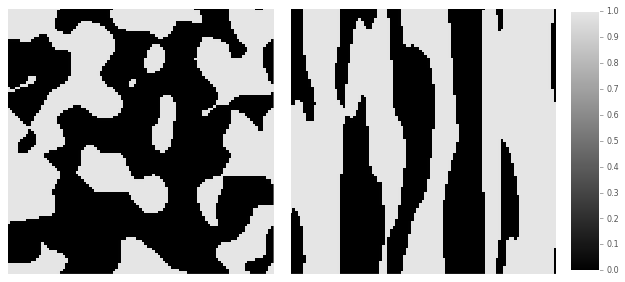We can compute the 2-point statistics for these two periodic microstructures using the correlate function from pymks.stats. This function computes all of the autocorrelations and cross-correlation(s) for a microstructure. Before we compute the 2-point statistics, we will discretize them using the PrimitiveBasis function.

Let’s take a look at the two autocorrelations and the cross-correlation for these two microstructures.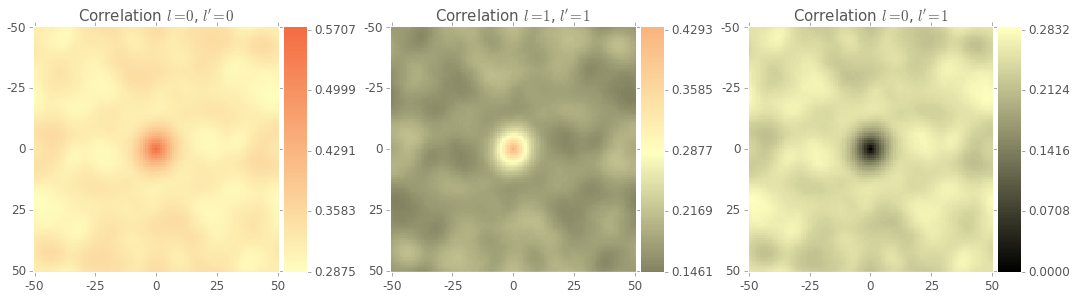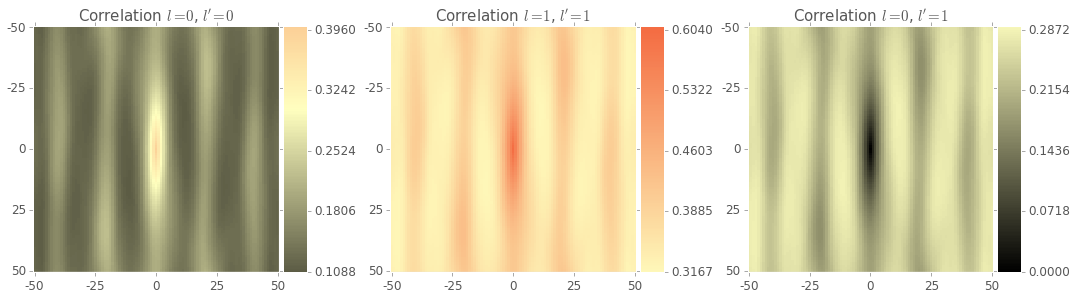2-Point statistics provide an object way to compare microstructures, and have been shown as an effective input to machine learning methods.

### Predict Homogenized Properties

In this section of the intro, we are going to predict the effective stiffness for two phase microstructures using the MKSHomogenizationModel, but we could have chosen any other effective material property.

First we need to make some microstructures and their effective stress values to fit our model. Let’s create 200 random instances 3 different types of microstructures, totaling to 600 microstructures.

Once again, X_train is our microstructures. Throughout PyMKS y is used as either the prpoerty or the field we would like to predict. In this case y_train is the effective stress values for X_train. Let’s look at one of each of the three different types of microstructures.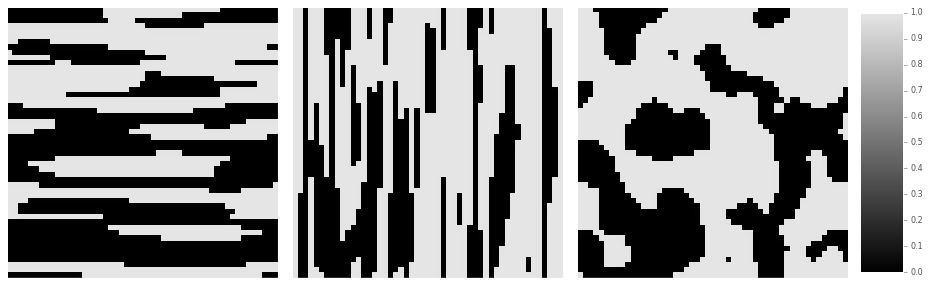The MKSHomogenizationModel uses 2-point statistics, so we need provide a discretization method for the microstructures by providing a basis function. We will also specify which correlations we want.

Let’s fit our model with the data we created.

Now let’s make some new data to see how good our model is.

We will try and predict the effective stress of our X_test microstructures.

The MKSHomogenizationModel generates low dimensional representations of microstructures and regression methods to predict effective properties. Take a look at the low dimensional representations.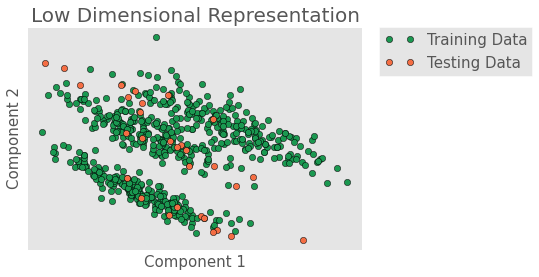Now let’s look at a goodness of fit plot for our MKSHomogenizationModel.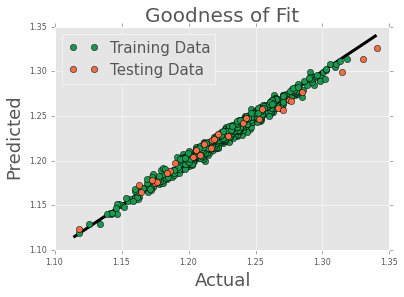Looks good.

The MKSHomogenizationModel can be used to predict effective properties and processing-structure evolutions.

### Predict Local Properties

In this section of the intro, we are going to predict the local strain field in a microstructure using MKSLocalizationModel, but we could have predicted another local property.

First we need some data, so let’s make some.

Once again, X_delta is our microstructures and y_delta is our local strain fields. We need to discretize the microstructure again so we will also use the same basis function.

Let’s use the data to fit our MKSLocalizationModel.

Now that we have fit our model, we will create a random microstructure and compute its local strain field using finite element analysis. We will then try and reproduce the same strain field with our model.

Let’s look at the microstructure and its local strain field.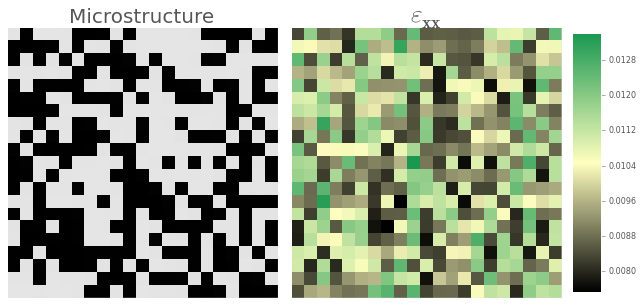Now let’s pass that same microstructure to our MKSLocalizationModel and compare the predicted and computed local strain field.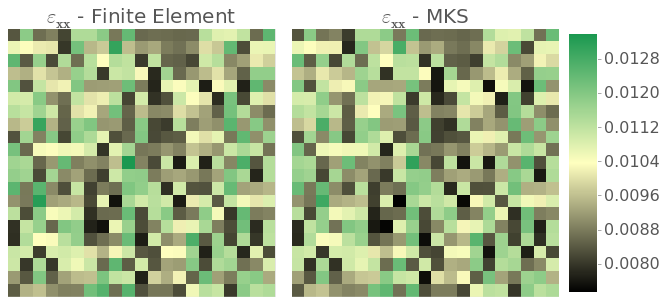Not bad.

The MKSLocalizationModel can be used to predict local properties and local processing-structure evolutions.